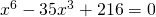# 10.5 Solving Quadratic Equations Using Substitution

Factoring trinomials in which the leading term is not 1 is only slightly more difficult than when the leading coefficient is 1. The method used to factor the trinomial is unchanged.

Example 10.5.1

Solve for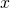in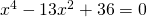.

First start by converting this trinomial into a form that is more common. Here, it would be a lot easier when factoring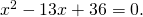There is a standard strategy to achieve this through substitution.

First, let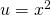. Now substitute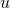for every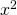, the equation is transformed into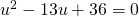.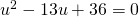factors into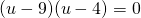.

Once the equation is factored, replace the substitutions with the original variables, which means that, since, thenbecomes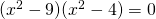.

To complete the factorization and find the solutions for, thenmust be factored once more. This is done using the difference of squares equation: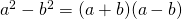.

Factoringthus leaves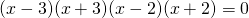.

Solving each of these terms yields the solutions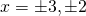.

This same strategy can be followed to solve similar large-powered trinomials and binomials.

Example 10.5.2

Factor the binomial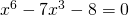.

Here, it would be a lot easier if the expression for factoring was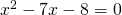.

First, let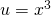, which leaves the factor of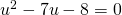.easily factors out to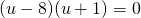.

Now that  the substituted values are factored out, replace thewith the original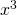. This turnsinto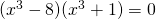.

The factored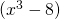and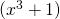terms can be recognized as the difference of cubes.

These are factored using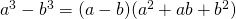and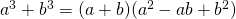.

And so,factors out to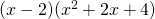andfactors out to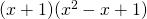.

Combining all of these terms yields: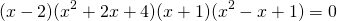The two real solutions are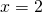and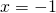. Checking for any others by using the discriminant reveals that all other solutions are complex or imaginary solutions.

# Questions

Factor each of the following polynomials and solve what you can.

1.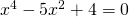2.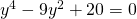3.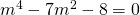4.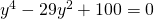5.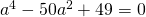6.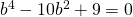7.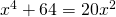8.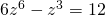9.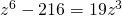10.